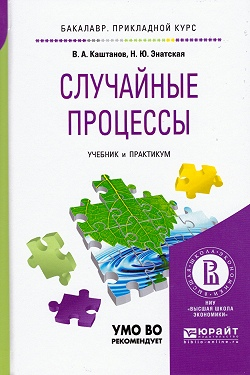• A
• A
• A
• ABC
• ABC
• ABC
• А
• А
• А
• А
• А
Regular version of the site

## Случайные процессы: учебник и практикум для прикладного бакалавриата

Юрайт, 2017.
Каштанов В. А., Энатская Н. Ю.

The textbook has passed practical tests and written on the basis of the readable authors for many years. Presented in textbook materials give students orientation in the solution of many practical problems in a number of areas, constitute the initial level to obtain a broader and deeper education in the field of probability theory. The book provides an overview of the theory of stochastic processes, detailed material on the theory of Markov processes with discrete time (Markov chains) and continuous-time. In addition to the solved problems for each Chapter of the textbook suggested problems to solve and theoretical questions to test the quality of the learning material.